The .Rmd file (code) for this replication file together with underlying data is available at: https://github.com/wzb-ipi/vaccine_solidarity

# 1 Setup

knitr::opts_chunk$set(echo=TRUE, warning=FALSE, message=FALSE, comment=NA) # options(qwraps2_markup = "markdown") ## Packages if (!require(pacman)) install.packages("pacman") ## Loading required package: pacman pacman::p_load( ggpubr, # for ggarrange cregg, # conjoint estimatr, haven, # load sav kableExtra, # Prettier RMarkdown (1.0.1) knitr, labelled, sjlabelled, stargazer, texreg, tidyverse ) # Whether to run from raw data prep_data <- FALSE # 2 Data preparation deal_labels <- c("No deal" =0, "20 give 20 bn" = 1, "40 give 20b" = 2, "20 give 40 bn" = 3, "40 give 40 bn" = 4) # vignettes values vign_values <- expand.grid(trading_importance = 0:1, risk=0:1, deal=0:4) %>% arrange(trading_importance, risk) %>% set_value_labels( trading_importance = c('Control: Wirtschaft bleibt unberührt'=0, 'Treatment: Wirtschaft schrumpft um 5%'=1), risk=c('Control: Kein Risiko durch Mutationen'=0, 'Treatment: Erhöhtes Risiko durch Mutationen'=1), deal= deal_labels) %>% mutate(vignr=1:20) %>% as_tibble() head(vign_values) |> kable(caption = "Conditions", format = "pipe") Conditions trading_importance risk deal vignr 0 0 0 1 0 0 1 2 0 0 2 3 0 0 3 4 0 0 4 5 0 1 0 6 if(prep_data){ main_df <- read_csv('1_input/combined.csv') %>% mutate( party = factor(party_id, 1:9, c("CDU.CSU", "CDU.CSU", "SPD", "AFD", "Greens", "FDP", "Left", "Other", "No party"))) %>% group_by(ID) %>% mutate(migration_background = mean(migration_background, na.rm = TRUE)) %>% ungroup() df_2 <- dplyr::filter(main_df, wave == 2) |> select(ID, solidarity_behaviour, solidarity_attitude, treatment_video, starts_with("c_0031"), starts_with("c_0032"), starts_with("vignette"), starts_with("conjoint_")) w4 <- main_df |> dplyr::filter(wave==4) |> select(group, ID, perspective_fed_indian, perspective_fed_german, vaccinated, party, migration_background, run1_exp8, exp7_money1, exp7_doses1, run2_exp8, exp7_money2, exp7_doses2) df_4 <- list( w4 %>% mutate(vignr = as.numeric(run1_exp8), round = 1) |> rename(cash = exp7_money1, doses = exp7_doses1), w4 %>% mutate(vignr = as.numeric(run2_exp8), round = 2) |> rename(cash = exp7_money2, doses = exp7_doses2) ) %>% bind_rows %>% left_join(vign_values) %>% dplyr::filter(group !=3) %>% mutate(id = ID, others_number = (deal==1 | deal == 3)*2 + (deal==2 | deal == 4)*4, others_giving = (deal==1 | deal == 2)*2 + (deal==3 | deal == 4)*4, others_average = others_giving/others_number, others_average = ifelse(others_number==0, 0, others_average), deal = factor(deal, deal_labels, names(deal_labels)), risk_factor = factor(risk, 0:1, c("Low", "High")), trading_factor = factor(trading_importance, 0:1, c("Low", "High")), risk = risk - mean(risk), trading_importance = trading_importance - mean(trading_importance), others_number_norm = others_number - mean(others_number), others_giving_norm = others_giving - mean(others_giving), cash_billions = cash*1000, cash_billions_log = log(1 + cash_billions)) |> left_join(df_2) df_4 |> write_rds("df_4.rds") df_2 |> write_rds("df_2.rds") } df_4 <- read_rds("df_4.rds") df_2 <- read_rds("df_2.rds") # 3 Numbers provided in Introduction (Vaccine background data from OWID) owd <- read.csv("1_input/owid-covid-data-20221007.csv") owd_world <- filter(owd, location == "World") owd <- owd |> dplyr::filter(continent != "") alt_date = "2022-10-01" survey_date = "2021-09-22" small_owd <- owd %>% dplyr::filter(date == survey_date) %>% select(iso_code, location, gdp_per_capita, population, people_fully_vaccinated_per_hundred, total_deaths_per_million, total_vaccinations) small_owd_alt <- owd %>% dplyr::filter(date == alt_date) %>% select(iso_code, location, gdp_per_capita, population, people_fully_vaccinated_per_hundred, total_deaths_per_million, total_vaccinations)  ## 3.1 Global vaccination owd_world |> filter(date %in% c(survey_date, alt_date)) |> select(date, people_fully_vaccinated_per_hundred, total_vaccinations) |> kable(caption = "Vaccination at the time of survey and most recently", format = "pipe") Vaccination at the time of survey and most recently date people_fully_vaccinated_per_hundred total_vaccinations 2021-09-22 32.18 6065656204 2022-10-01 62.71 12774122923 ## 3.2 Historical distribution as explained by GDP and by deaths r2s <- c( r2_gdp = lm_robust( people_fully_vaccinated_per_hundred ~ gdp_per_capita, data = small_owd)$r.squared,

r2_deaths = lm_robust(
people_fully_vaccinated_per_hundred ~  total_deaths_per_million,
data = small_owd)$r.squared, r2_deaths_most_recent = lm_robust( people_fully_vaccinated_per_hundred ~ total_deaths_per_million, data = small_owd_alt)$r.squared)

data.frame(var = names(r2s), R2 = r2s)|> kable(format = "pipe")
var R2
r2_gdp r2_gdp 0.5633888
r2_deaths r2_deaths 0.0778412
r2_deaths_most_recent r2_deaths_most_recent 0.0344874

## 3.3 Coverage in top 10 and bottom 10 countries (with data and population > 500,000)

small_owd |>
arrange(people_fully_vaccinated_per_hundred) |>
filter(!is.na(people_fully_vaccinated_per_hundred) &
population > 500000) |>
mutate(N = n()) |>
select(location, people_fully_vaccinated_per_hundred, N) |>
slice(10, (n()-9)) %>%
kable(caption = "Most and least vaccinated", format = "pipe")
Most and least vaccinated
location people_fully_vaccinated_per_hundred N
Kenya 1.64 102
Chile 72.28 102
small_owd_alt |>
arrange(people_fully_vaccinated_per_hundred) |>
filter(!is.na(people_fully_vaccinated_per_hundred) &
population > 500000) |>
mutate(N = n()) |>
select(location, people_fully_vaccinated_per_hundred, N) |>
slice(10, (n()-9)) %>%
kable(caption = "Most and least vaccinated, most recent---note few countries reporting", format = "pipe")
Most and least vaccinated, most recent—note few countries reporting
location people_fully_vaccinated_per_hundred N
Israel 66.24 36

### 3.3.1 Assessed shares

pc <- 80000000000/ (small_owd %>% dplyr::filter(gdp_per_capita > 27000) %>% pull(population) %>% sum)

small_owd <- small_owd %>% mutate(
bill = ifelse(gdp_per_capita > 27000, pc*population, 0)
)

small_owd %>%
dplyr::filter(location == "Germany" | location == "United States" ) %>%
kable(format = "pipe")
iso_code location gdp_per_capita population people_fully_vaccinated_per_hundred total_deaths_per_million total_vaccinations bill
DEU Germany 45229.25 83408554 63.26 1116.468 108284950 5818540801
USA United States 54225.45 336997624 56.73 2016.174 395249407 23508792935

# 4 Analysis: Raw patterns

# Support sizes

amounts_d <- seq(0, 22, .5)
amounts_v <- seq(0, 200, 1)

# Figures cumulative distribution
support <- function(x = df_4$cash_billions, amounts = amounts_d) data.frame( amounts = amounts, support = sapply(amounts, function(j) mean(x >= j, na.rm = TRUE))) s1 <- list( Low = support( df_4 %>% dplyr::filter(risk_factor == "Low" & trading_factor == "Low") %>% pull(cash_billions), amounts = amounts_d), High = support( df_4 %>% dplyr::filter(risk_factor == "High" & trading_factor == "High")%>% pull(cash_billions), amounts = amounts_d)) %>% bind_rows(.id = "Costs") s2 <- list( Low = support( df_4 %>% dplyr::filter(deal == "No deal") %>% pull(cash_billions)), High = support( df_4 %>% dplyr::filter(deal == "40 give 40 bn") %>% pull(cash_billions))) %>% bind_rows(.id = "Multilateralism") s3 <- list( Low = support( df_4 %>% dplyr::filter(risk_factor == "Low" & trading_factor == "Low") %>% pull(doses), amounts_v), High = support( df_4 %>% dplyr::filter(risk_factor == "Low" & trading_factor == "High") %>% pull(doses), amounts_v)) %>% bind_rows(.id = "Costs") s4 <- list( Low = support( df_4 %>% dplyr::filter(deal == "No deal") %>% pull(doses), amounts_v), High = support( df_4 %>% dplyr::filter(deal == "40 give 40 bn") %>% pull(doses), amounts_v)) %>% bind_rows(.id = "Multilateralism") # 5 Figure 1: Distribution of support for contributions of different sizes supports <- ggarrange( s1 %>% ggplot(aes(amounts, support, color = Costs)) + geom_line() + ylim(0, 1) + theme_bw() + xlab("German contribution (bn Euro)") + ylab("Share supporting") + theme(legend.position = "bottom"), s2 %>% ggplot(aes(amounts, support, color = Multilateralism)) + geom_line() + ylim(0, 1) + theme_bw() + xlab("German contribution (bn Euro)") + ylab("Share supporting") + theme(legend.position = "bottom") + ylab(""), s3 %>% ggplot(aes(amounts, support, color = Costs)) + geom_line() + ylim(0, 1) + theme_bw() + xlab("German contribution (mio vaccines)") + ylab("Share supporting") + theme(legend.position = "bottom"), s4 %>% ggplot(aes(amounts, support, color = Multilateralism)) + geom_line() + ylim(0, 1) + theme_bw() + xlab("German contribution (mio vaccines)") + ylab("Share supporting") + theme(legend.position = "bottom") + ylab(""), nrow = 2, ncol = 2 ) pdf("2_output/cumulative.pdf", height = 6, width = 10) supports dev.off() png 2  supports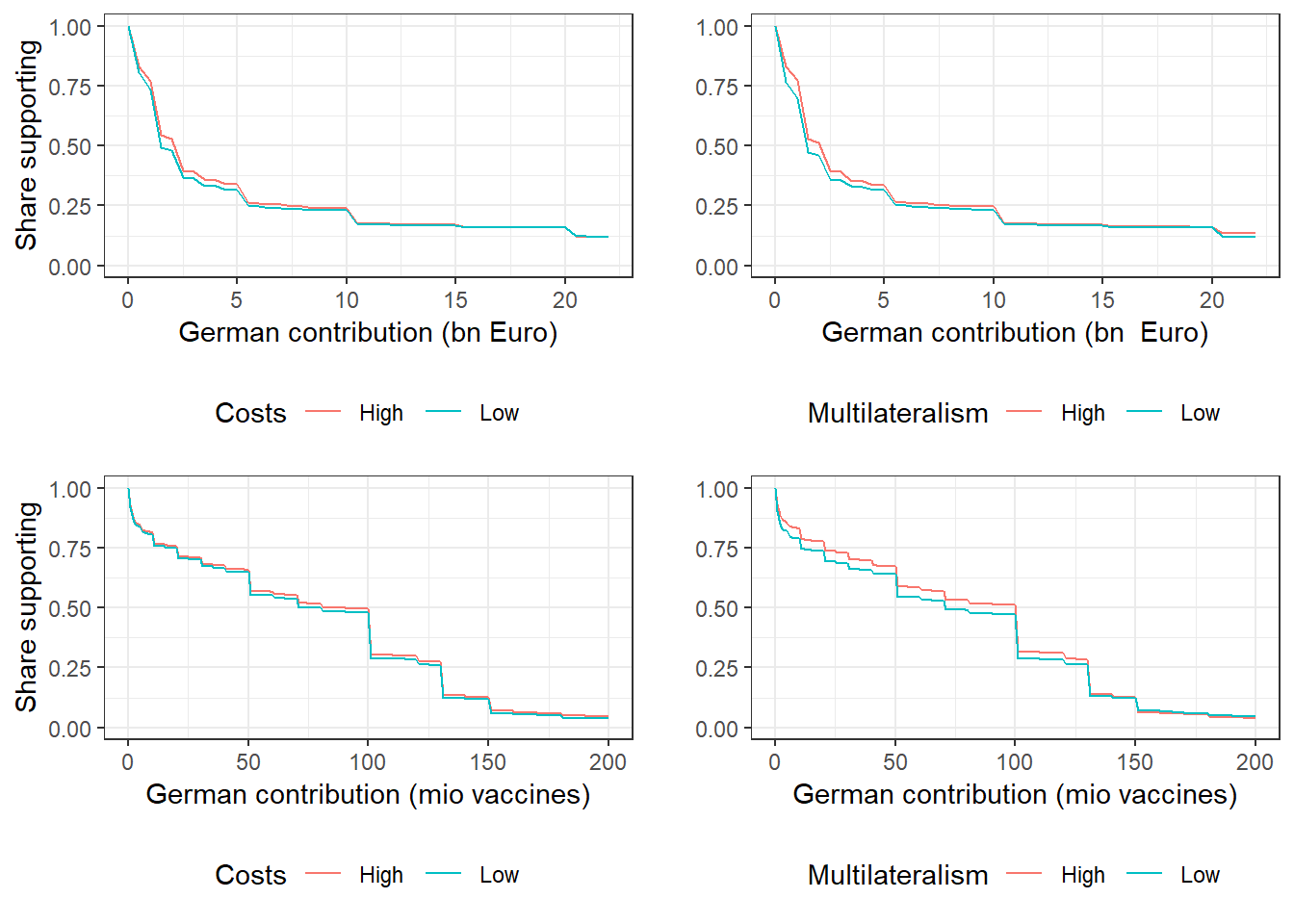Basic variation by vaccination status df_4 %>% group_by(vaccinated) %>% summarize(median_cash = median(cash_billions), mean_cash = mean(cash_billions), median_doses = median(doses), mean_doses = mean(doses), ) |> kable(format = "pipe") vaccinated median_cash mean_cash median_doses mean_doses 0 1 8.387918 50 64.92555 1 2 8.724218 100 80.48265 main_results <- list( cash = lm_robust(cash_billions ~ trading_importance*risk*others_number_norm*others_giving_norm + round, fixed_effects = ~id, se_type = "stata", data = df_4) %>% tidy, doses = lm_robust(doses ~ trading_importance*risk*others_number_norm*others_giving_norm + round, fixed_effects = ~id, se_type = "stata", data = df_4) %>% tidy) %>% bind_rows() treatments <- c("trading_importance", "risk", "others_number", "others_giving") treatments_norm <- c("trading_importance", "risk", "others_number_norm", "others_giving_norm") treatment_labels <- c("Trading importance", "Risk", "Number of others giving (10s)", "Amount given by other countries\n(10s of billions)") Basic variation by risk factor df_4 %>% group_by(risk_factor) %>% summarize( median_cash = median(cash_billions), mean_cash = mean(cash_billions), median_doses = median(doses), mean_doses = mean(doses)) |> kable(format = "pipe") risk_factor median_cash mean_cash median_doses mean_doses Low 1.15 8.346641 80 76.06485 High 2.00 8.994930 100 79.93953 # 6 Figure 2: Marginal effects of conditions The average proposal is: 8.67 billion Euros and 78 million doses. The median proposal is: 2 billion Euros and 90 million doses. These are close to actual https://www.auswaertiges-amt.de/en/aussenpolitik/themen/gesundheit/covax/2396914. The median in risk conditions is proposal is: 2 billion Euros and 100 million doses. figure_2 <- main_results %>% dplyr::filter(term %in% treatments_norm) %>% mutate(Treatment = factor(term, treatments_norm, treatment_labels), outcome = factor(outcome, c("cash_billions", "doses"), c("Cash (billion Euros)", "Doses (Millions)"))) %>% ggplot(aes(estimate, Treatment)) + geom_point()+ geom_errorbar(aes(xmin = conf.low, xmax = conf.high), width = .1)+ geom_vline(xintercept=0, linetype="longdash", lwd=0.35, colour = "#B55555") + theme_bw() + facet_grid(~outcome, scales = "free_x")+ ylab("") df_mean <- df_4 %>% group_by(risk_factor) %>% summarize(average = mean(cash_billions, na.rm = TRUE)) %>% ungroup() figure_2_1 <- df_4 %>% ggplot(aes(risk_factor, cash_billions)) + geom_boxplot() + ylab("Billions of Euros") + xlab("Risk") + scale_y_sqrt() + geom_point(data = df_mean, mapping = aes(x = risk_factor, y = average), color="red") figure_2_2 <- df_4 %>% ggplot(aes(risk_factor, doses)) + geom_boxplot() + ylab("Millions of doses") + xlab("Risk") figure_2_1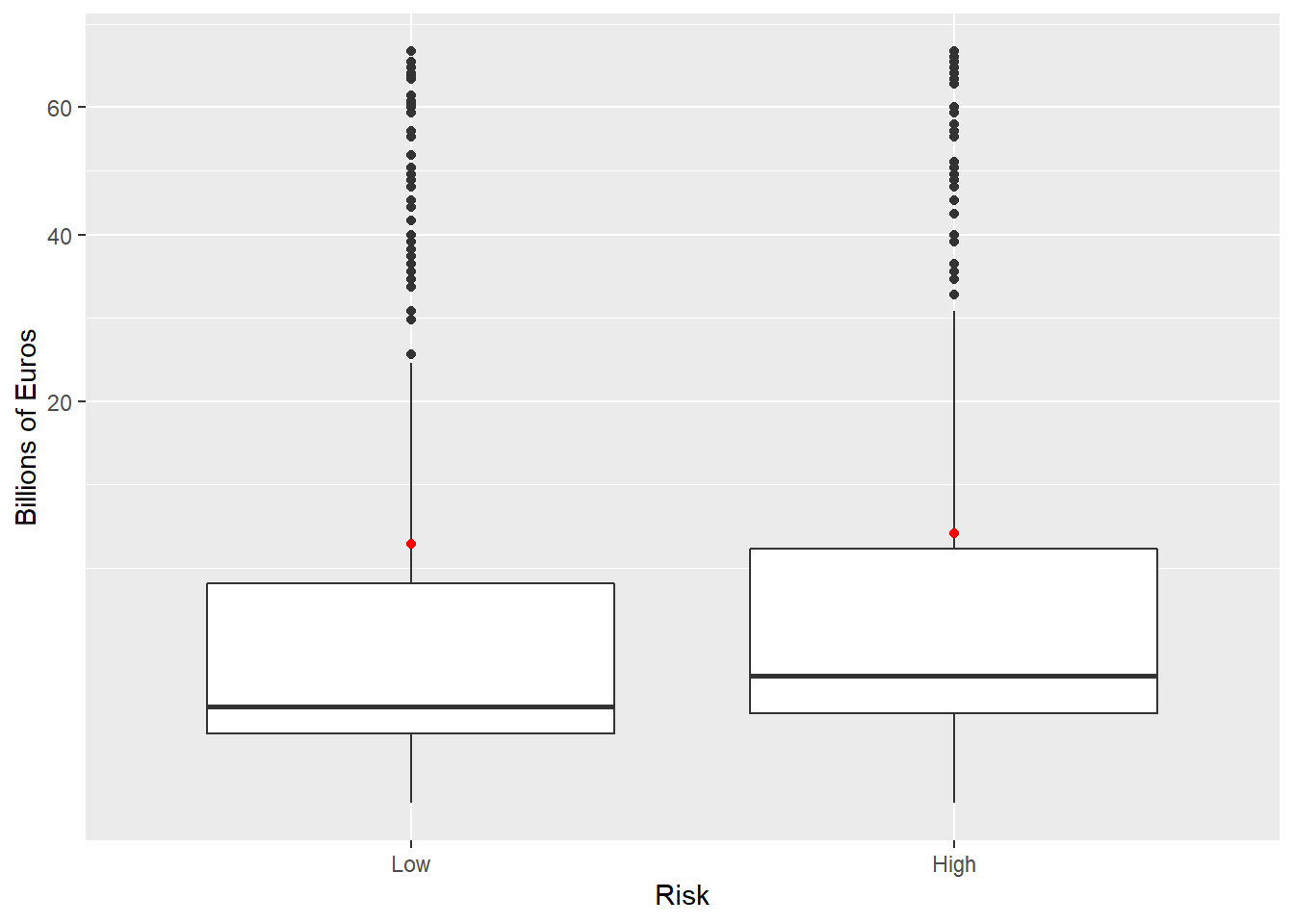figure_2_2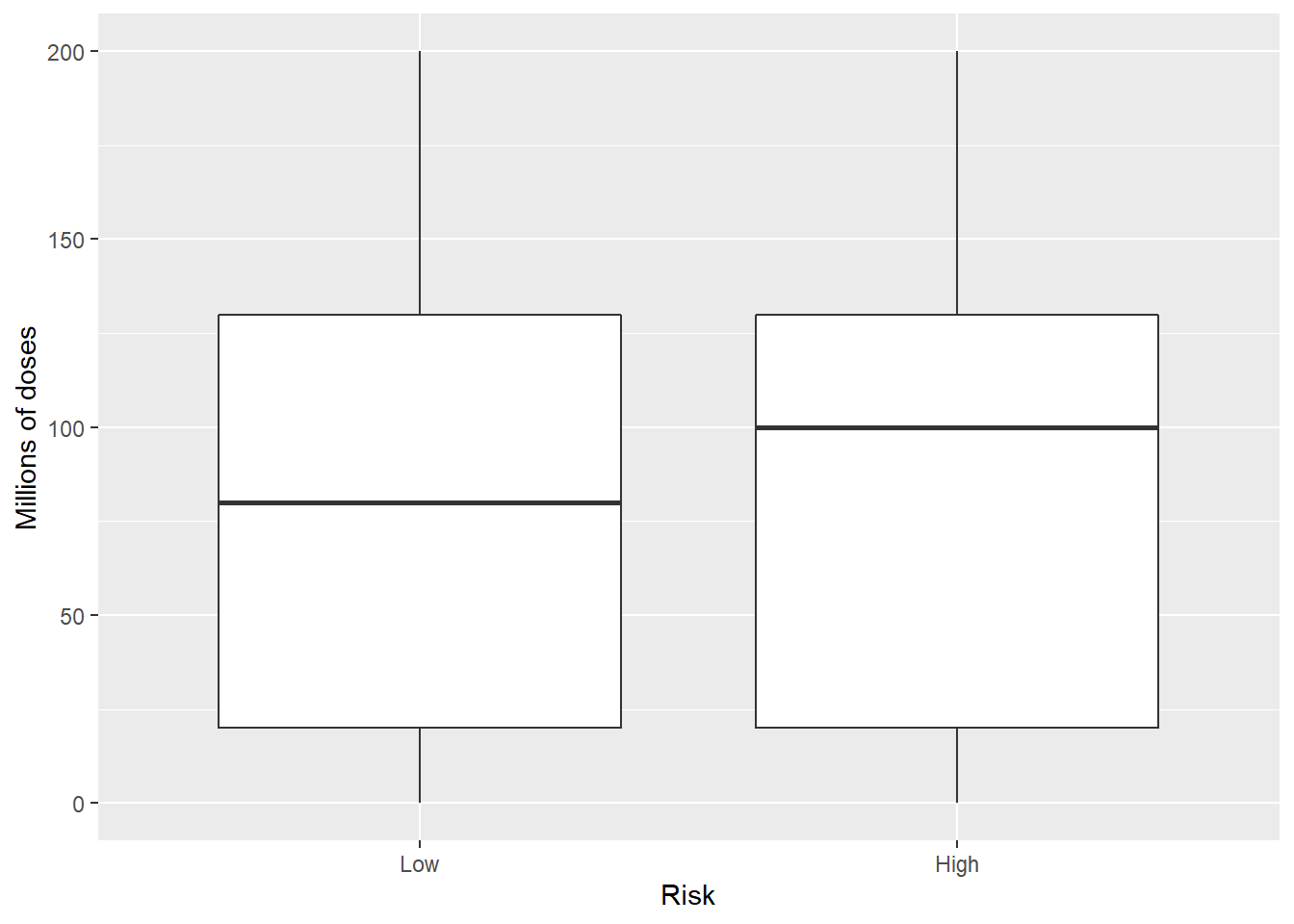Note that the doses options was limited to between 0 and 200 m. Offers are responsive to risk and economic impacts but not much to coordination considerations. figure_2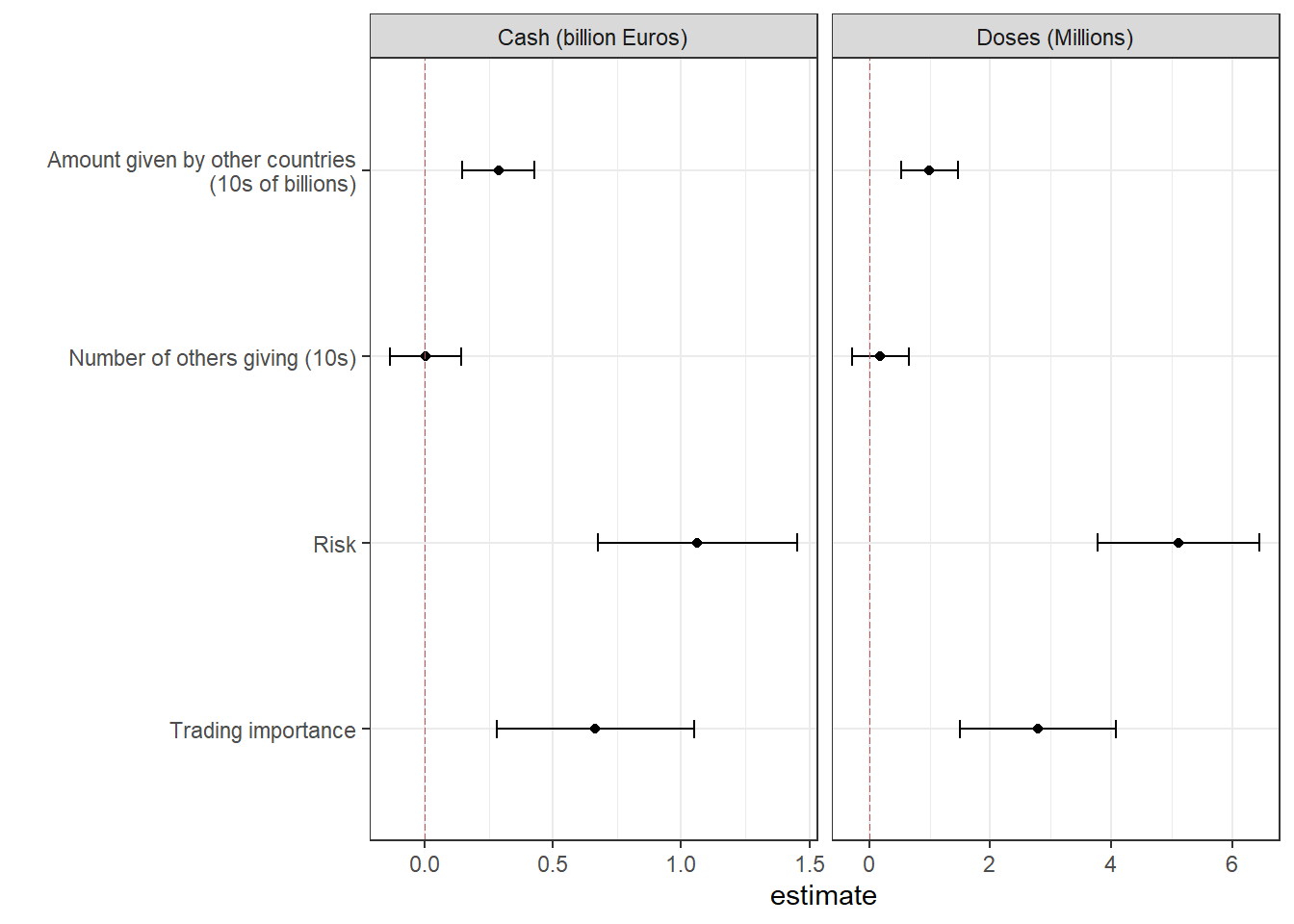pdf("2_output/E1_main.pdf", width = 6, height = 3) figure_2 dev.off() png 2  Trading importance and risk are substitutes for cash but not doses. Without demeaning: lm_robust(cash_billions ~ trading_factor*risk_factor+ round, fixed_effects = ~id, se_type = "stata", data = df_4) %>% tidy %>% kbl(digit =2) %>% kable_minimal() term estimate std.error statistic p.value conf.low conf.high df outcome trading_factorHigh 0.83 0.23 3.54 0.00 0.37 1.29 10521 cash_billions risk_factorHigh 1.64 0.24 6.94 0.00 1.18 2.11 10521 cash_billions round 0.01 0.12 0.10 0.92 -0.22 0.24 10521 cash_billions trading_factorHigh:risk_factorHigh -0.65 0.32 -2.01 0.04 -1.28 -0.02 10521 cash_billions Logging helps and substitution not as strong: lm_robust(log(cash_billions+1) ~ trading_factor*risk_factor+ round, fixed_effects = ~id, se_type = "stata", data = df_4) %>% tidy %>% kbl(digit =2) %>% kable_minimal() term estimate std.error statistic p.value conf.low conf.high df outcome trading_factorHigh 0.10 0.01 6.76 0.00 0.07 0.13 10521 log(cash_billions + 1) risk_factorHigh 0.16 0.01 10.80 0.00 0.13 0.19 10521 log(cash_billions + 1) round 0.02 0.01 2.10 0.04 0.00 0.03 10521 log(cash_billions + 1) trading_factorHigh:risk_factorHigh -0.05 0.02 -2.34 0.02 -0.09 -0.01 10521 log(cash_billions + 1) ## 6.1 Full results from saturated models main_results %>% kbl(digit =2) %>% kable_minimal() term estimate std.error statistic p.value conf.low conf.high df outcome trading_importance 0.67 0.20 3.38 0.00 0.28 1.05 10509 cash_billions risk 1.06 0.20 5.37 0.00 0.68 1.45 10509 cash_billions others_number_norm 0.00 0.07 0.05 0.96 -0.14 0.14 10509 cash_billions others_giving_norm 0.29 0.07 4.01 0.00 0.15 0.43 10509 cash_billions round 0.02 0.12 0.17 0.86 -0.21 0.25 10509 cash_billions trading_importance:risk -0.23 0.39 -0.60 0.55 -1.00 0.53 10509 cash_billions trading_importance:others_number_norm 0.03 0.15 0.19 0.85 -0.26 0.32 10509 cash_billions risk:others_number_norm 0.05 0.14 0.35 0.73 -0.23 0.33 10509 cash_billions trading_importance:others_giving_norm -0.03 0.15 -0.24 0.81 -0.32 0.25 10509 cash_billions risk:others_giving_norm -0.04 0.15 -0.24 0.81 -0.33 0.26 10509 cash_billions others_number_norm:others_giving_norm -0.02 0.04 -0.43 0.67 -0.11 0.07 10509 cash_billions trading_importance:risk:others_number_norm -0.30 0.28 -1.08 0.28 -0.86 0.25 10509 cash_billions trading_importance:risk:others_giving_norm -0.02 0.30 -0.06 0.95 -0.60 0.56 10509 cash_billions trading_importance:others_number_norm:others_giving_norm -0.12 0.08 -1.38 0.17 -0.28 0.05 10509 cash_billions risk:others_number_norm:others_giving_norm 0.17 0.09 2.04 0.04 0.01 0.34 10509 cash_billions trading_importance:risk:others_number_norm:others_giving_norm -0.29 0.17 -1.68 0.09 -0.62 0.05 10509 cash_billions trading_importance 2.78 0.66 4.24 0.00 1.50 4.07 10509 doses risk 5.11 0.68 7.52 0.00 3.78 6.44 10509 doses others_number_norm 0.18 0.24 0.76 0.45 -0.29 0.65 10509 doses others_giving_norm 1.00 0.24 4.22 0.00 0.53 1.46 10509 doses round 1.80 0.38 4.72 0.00 1.05 2.55 10509 doses trading_importance:risk -0.67 1.24 -0.54 0.59 -3.10 1.76 10509 doses trading_importance:others_number_norm 0.30 0.48 0.63 0.53 -0.64 1.24 10509 doses risk:others_number_norm 0.48 0.48 1.02 0.31 -0.45 1.42 10509 doses trading_importance:others_giving_norm 0.01 0.47 0.02 0.99 -0.92 0.93 10509 doses risk:others_giving_norm -0.58 0.48 -1.20 0.23 -1.51 0.36 10509 doses others_number_norm:others_giving_norm -0.38 0.14 -2.68 0.01 -0.65 -0.10 10509 doses trading_importance:risk:others_number_norm 0.09 0.95 0.09 0.93 -1.78 1.96 10509 doses trading_importance:risk:others_giving_norm 0.34 0.97 0.35 0.73 -1.56 2.23 10509 doses trading_importance:others_number_norm:others_giving_norm -0.24 0.27 -0.91 0.37 -0.77 0.28 10509 doses risk:others_number_norm:others_giving_norm 0.36 0.28 1.28 0.20 -0.19 0.92 10509 doses trading_importance:risk:others_number_norm:others_giving_norm -0.85 0.54 -1.57 0.12 -1.91 0.21 10509 doses # 7 Table 2: Structural Analysis We assume: $u = (a + bT + cR) \log(Y + x) - x^2 - g(x - ky)^2$ where $$T$$ is trading importance, $$R$$ is health risk, $$Y$$ is total Amount given by other countries and $$y$$ is average Amount given by other countries First order condition for maximization is: $\frac{(a + bT + cR)}{ (Y + x)} - 2x - 2g(x - ky) = 0$ This yields the following quadratic. We take the second solution (with positive contributions). $- 2(1+g)x^2- 2((1+g)Y - gky)x +(2gkyY+(a + bT + cR)) = 0$ # Quadratic solution maxx <- function(a,b,c,g,k,ZT,ZR,ZY,Zy) { AA = -2*(1+g) BB = -2*((1+g)*ZY - g*k*Zy) CC = 2*g*k*Zy*ZY + a+b*ZT+c*ZR (-BB - (BB^2 - 4*AA*CC)^.5)/(2*AA) } maxx(a=1,b=1,c=1,g=1, k=1,ZT=1,ZR=1,ZY=1,Zy=1)  0.8956439 lik_x <- function(x, sigma, a,b,c,g,k,ZT,ZR,ZY,Zy) dnorm(x, maxx(a, b, c, g, k, ZT, ZR, ZY, Zy), sd = sigma) # Estimation est <- function(data){ LL <- function(a=1,b=1,c=1,g=1,k=1, sigma=1) { R <- with(data, lik_x(x, sigma, a, b, c, g, k, ZT = trading_importance, ZR = risk, ZY = others_giving, Zy = others_average)) -sum(log(R)) } M <- bbmle::mle2( LL, # method = "L-BFGS-B", optimizer = "nlminb", start = list(a = 1, b = 1, c = 1, g = 2, k = 1, sigma = .05), lower = list(a = -20, b = -10, c = -20, g = .01, k = -10, sigma = .02), upper = list(a = 20, b = 10, c = 10, g = 10, k = 10, sigma = 10)) # Format output from estimation out <- bbmle::coef(bbmle::summary(M)) %>% data.frame() names(out) <- c("estimate", "std.error", "statistic", "p.value") out %>% mutate(conf.low = estimate - 1.96*std.error, conf.high = estimate + 1.96*std.error) } Note structural estimates do not include fixed effects. # Note that starting values were found using a grid search to approximate the region of the solution df_4 <- df_4 %>% mutate(x = cash_billions) LL <- function(a=1,b=1,c=1,g=1,k=1, sigma=1) { R <- with(df_4, lik_x(x, sigma, a, b, c, g, k, ZT = trading_importance, ZR = risk, ZY = others_giving, Zy = others_average)) -sum(log(R)) } # .a .b .c .g .k .sigma # 243. -10 36.7 0.667 5 16 M <- bbmle::mle2( LL, # method = "L-BFGS-B", optimizer = "nlminb", start = list(a = 240, b = -11, c = 41, g = .8, k = 4, sigma = 16), lower = list(a = 0, b = -20, c = -60, g = .01, k = -10, sigma = .02), upper = list(a = 400, b = 20, c = 60, g = 5, k = 10, sigma = 30)) # Format output from estimation out <- bbmle::coef(bbmle::summary(M)) %>% data.frame() names(out) <- c("estimate", "std.error", "statistic", "p.value") # Flag: Should be able to do better ci's than this out %>% mutate(conf.low = estimate - 1.96*std.error, conf.high = estimate + 1.96*std.error) %>% kable(format = "pipe", digits = 2) estimate std.error statistic p.value conf.low conf.high a 240.62 2.01 119.73 0.00 236.68 244.56 b -10.32 12.71 -0.81 0.42 -35.23 14.59 c 37.79 12.45 3.04 0.00 13.40 62.19 g 0.61 0.07 9.22 0.00 0.48 0.75 k 5.22 0.40 13.06 0.00 4.44 6.00 sigma 15.98 0.08 205.18 0.00 15.83 16.14 fileConn <- file("2_output/structural.tex") writeLines( out %>% mutate(parameter = c("$\\alpha$", "$\\beta$", "$\\delta$", "$\\gamma$", "$\\kappa$", "$\\sigma\$")) %>%
relocate(parameter) %>%
mutate(conf.low = estimate - 1.96*std.error,
conf.high = estimate + 1.96*std.error) %>%

kable(format = "latex",
caption = "Structural parameter estimates",
digits = 2,
booktabs = TRUE,
label = "structural", row.names = FALSE,
escape = FALSE,
align = c("c", "r", "r", "r", "r", "r", "r")) %>%
kable_styling(latex_options = "hold_position"),

fileConn)

close(fileConn)

# 8 Figure 3: Effect of video treatment on individual solidarity

outcomes <- c("solidarity_behaviour", "solidarity_attitude")
outcome_labels <- c("Solidarity Behavior", "Solidarity Attitude")
w2_treatments <- c("treatment_video")
w2_treatment_labels <- c("Treatment")
df_2 %>% group_by(treatment_video, solidarity_behaviour) |>
summarize(n = n()) |>
filter(!is.na(solidarity_behaviour)) |>
ungroup() |>
group_by(treatment_video) |>
mutate(share = n / sum(n)) |>
kable(caption = "Raw data", format = "pipe")
Raw data
treatment_video solidarity_behaviour n share
0 0.0 1821 0.2874961
0 0.2 537 0.0847805
0 0.4 1185 0.1870856
0 0.6 511 0.0806757
0 0.8 354 0.0558889
0 1.0 1926 0.3040733
1 0.0 1592 0.2600457
1 0.2 483 0.0788958
1 0.4 1070 0.1747795
1 0.6 477 0.0779157
1 0.8 383 0.0625613
1 1.0 2117 0.3458020
#    keep           donate UNICEF
#1  0 Mingle Points 75 Mingle Points
#2 10 Mingle Points 60 Mingle Punkte
#3 20 Mingle Points 45 Mingle Points
#4 30 Mingle Points 30 Mingle Points
#5 40 Mingle Points 15 Mingle Points
#6 50 Mingle Points 0 Mingle Points

hist1<-df_2 %>%
ggplot( aes(x = solidarity_behaviour)) +
geom_histogram()+
theme_bw() + facet_grid(~treatment_video)

hist2<-df_2 %>%
ggplot( aes(x = solidarity_attitude)) +
geom_histogram()+
theme_bw()  + facet_grid(~treatment_video)

hist1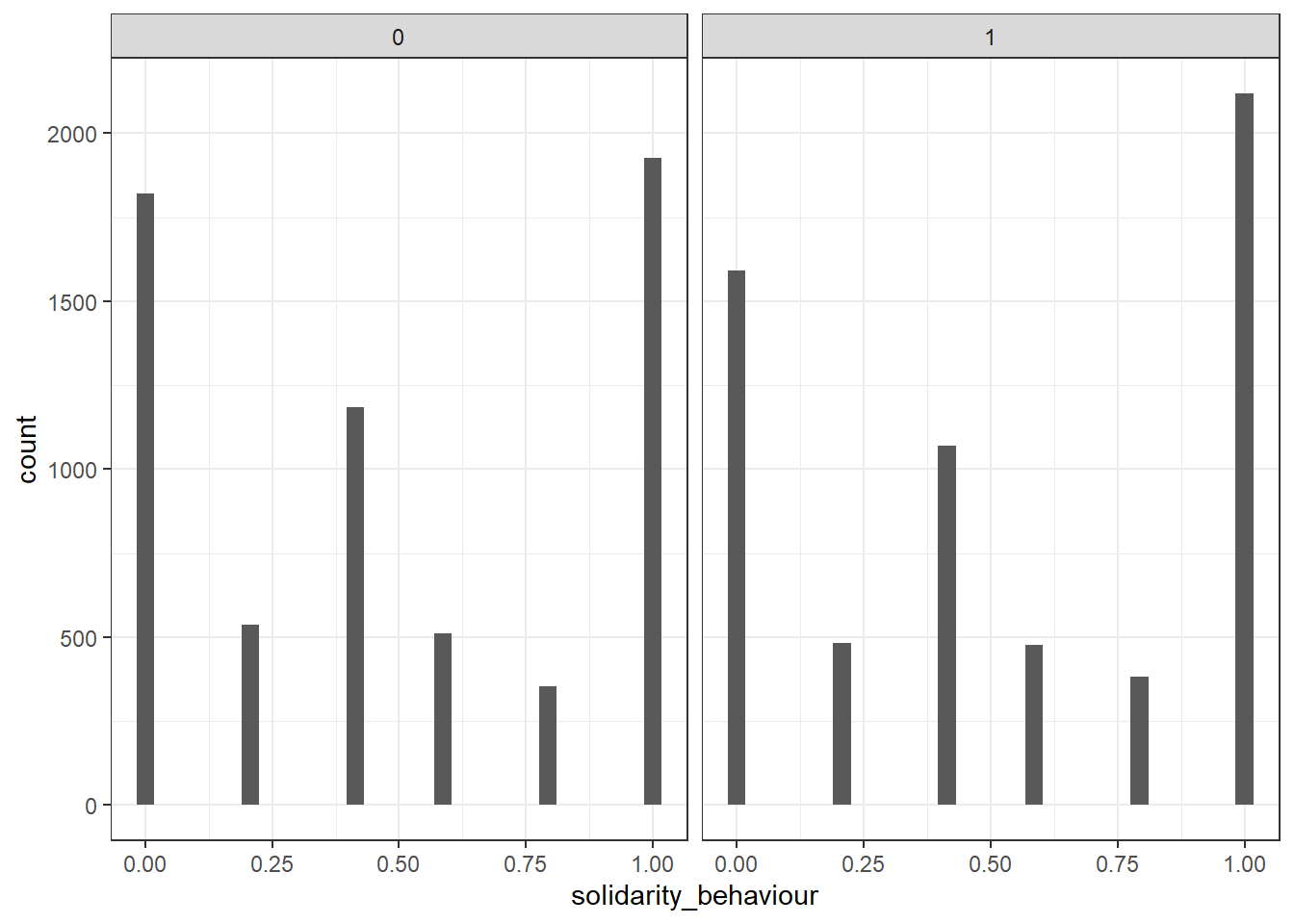hist2